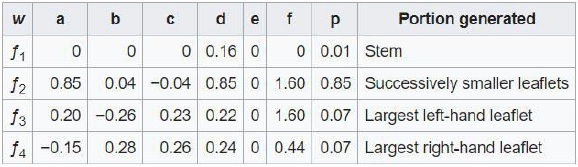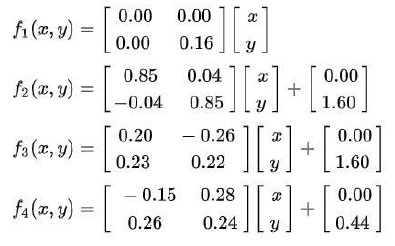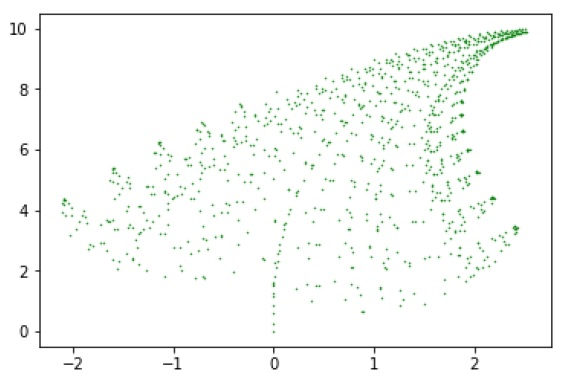# Barnsley Fern in Python

In this tutorial, we are going to learn about the Barnsley Fern, which is created by Michael Barnsley. The features of Barnsley Fern is similar to the fern shape. It is created by iterating over the four mathematical equations known as Iterated Function System(IFS). The transformation has the following formula.

f(x,y)=$$\begin{bmatrix}a & b \c & d \end{bmatrix}\begin{bmatrix}x \y \end{bmatrix}+\begin{bmatrix}e \f \end{bmatrix}$$

Source − Wikipedia

The values of the variables are −Source − Wikipedia

The four equation that Barnsley Fern proposed are −Source − Wikipedia

Now, we will see code to create the fern shape in Python.

### Example

# importing matplotlib module for the plot
import matplotlib.pyplot as plot
# importing random module to generate random integers for the plot
import random
# initialising the lists
x = 
y = 
# initialising a variable to zero to track position
current = 0
for i in range(1, 1000):
# generating a random integer between 1 and 100
z = random.randint(1, 100)
# checking the z range and appending corresponding values to x and y
# appending values to the x and y
if z == 1:
x.append(0)
y.append(0.16 * y[current])
if z >= 2 and z <= 86:
x.append(0.85 * x[current] + 0.04 * y[current])
y.append(-0.04 * x[current] + 0.85 * y[current] +1.6)
if z>= 87 and z<= 93:
x.append(0.2 * x[current] - 0.26 * y[current])
y.append(0.23 * x[current] + 0.22*(y[current])+1.6)
if z >= 94 and z <= 100:
x.append(-0.15 * x[current] + 0.28 * y[current])
y.append(0.26 * x[current] + 0.24 * y[current] + 0.44)
# incrementing the current value
current += 1
# plotting the graph using x and y
plot.scatter(x, y, s = 0.2, edgecolor = 'green')
plot.show()

### Output

If you run the above code, you will get the following result.## Conclusion

If you have any doubts in the tutorial, mention them in the comment section. Reference −Wikipedia